# Query Processing¶

## Overview¶

• SQL queries are converted bag relational algebra queries to be implemented
• We will investigate how different relational algebra operations are implemented
• We will see potentially multiple ways to implement each operation
• Overall picture of the DBMS system components is seen below. We are interested in the query execution component.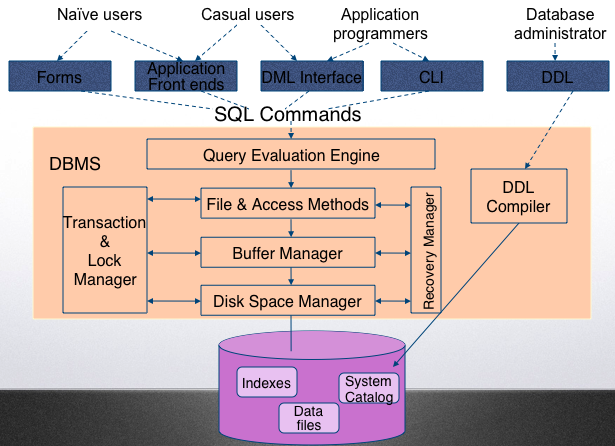## Disk Access Process (Overly Simplifed)¶

• Remember that to process any data, it must be first brought to memory.

• Some DBMS component indicates it wants to read record R

• File Manager:

Does security check

Uses access structures to determine the page it is on

Asks the buffer manager to find that page

• Buffer Manager

Checks to see if the page is already in the buffer

If so, gives the buffer address to the requestor

If not, allocates a buffer frame

Asks the Disk Manager to get the page

• Disk Manager

Determines the physical address(es) of the page

Asks the disk controller to get the appropriate block of data from the physical address

Disk controller instructs disk driver to do the dirty job

## Cost and Resources¶

• An SQL query is translated to a combination of relational algebra operations.

• Each operation in the query is given some resources: memory blocks to use for the operation.

We will use M to denote the number of blocks available to a query.

• We are interested in the overall cost of a query. We will only consider total number of disk pages read/written to execute the query.

We will use PAGES(R) to denote the total number of pages in relation R.

• Note that CPU complexity of a query is going to be disregarded (i.e. the complexity of the algorithm once the data is in memory) due to the following reasons:

• The cost of reading/writing data is much higher than any operation in memory. So, the donimating cost is disk access.
• We assume each algorithm is implemented as efficiently as possible.
• It may not even be possible to bring all the data for a query in memory at once, which may result in the data being read multiple times.
• The cost of a query (total number of pages read and written) depends on how much memory is available (M). We will see how these two are related for each operation.

## Iterator Interface¶

• Each operator in the database is implemented using three main functions:
• open() initializes the necessary memory structures (i.e. M buffers) and/or streams
• getNext() reads data input streams and processes the data until a block full of output is produced or the input is completely processed, puts the output to the output buffer
• close() frees all the structures used by the operator
• Since each operator works the same way, we can chain up the operators by using the input buffer for an operation as the output buffer for the operation below.
• If this is the last operation in the query tree, then the output buffer is simply the standard output to the user executing the query

## Iterator Example¶

• Suppose we are processing

```select * from R where C
```

by scanning the relation R.

• SCAN(R,C):

• open() reads the location of the data pages for R and allocates the necessary memory blocks (at least M=1 block is needed)
• getNext() reads blocks of R, for each tuple, if it satisfies the condition C, moves it to the output buffer until the output block is full and then copies the output block to the output stream
• close() frees all the memory used for this operation.

## Operator classes¶

• Query operators are classified into classes:

• One pass
• Two pass
• Multi-pass

depending on the availability of memory, storage method of the relation (i.e. sortedness for example) and the number of pages it occupies on disk.

## One pass algorithms¶

• The algorithms require one pass over a given relation.
• Note that this depends on the availability of the necessary amount of memory as explained for each operator.

## Duplicate removal¶

• Given M pages of memory. Let X be 1 page, and Y be M-1 pages in memory.

```Read R into X, 1 page at a time.

For each tuple t, check:
If tuple t is in Y:
it is already seen, remove t.
Else
insert t into Y.
```
• getNext() will read a one block from Y and output. The next time it will be called, we need to process the operation until another block in Y is filled.

• This is a one-pass operation only if after duplicate removal, R fits in M-1 blocks.

## Group by¶

• Given M pages of memory. Let X be 1 page, and Y be M-1 pages in memory.

```For each group in Y, we are going to keep:
the grouping attributes
the aggregate value
for min, max, count, sum: keep min, max, count,
sum of the tuples seen so far

for avg, keep count and sum.

Read R into X, 1 page at a time.
For each tuple t, check:
If the corresponding group for tuple t is in Y:
update its aggregates.

Else
create a new group for t into Y and initialize its statistics.
```
• This is possible only if all the results fit in Y (M-1) blocks. We cannot output the tuples until we finish processing all of R.

## Set and bag operators¶

• Bag Union of R and S:

```Read R one block at a time and output
Read S one block at a time and output
```
• Set Union of R and S:

```Read R and remove duplicates
Read S into the same space and continue to remove duplicates
```
• Set intersection:

```Read R, remove duplicates and store into M-1 blocks (section Y).
If the tuple is in Y
output and remove from Y
Else
```
• Bag Intersection:

Bag intersection requires that we keep track of how many copies of each tuple there are.

```Read R, group by all attributes and add a count for R.
Store into M-1 blocks (Y section).

Read S, for each tuple:
If is in the Y section
increment the count for S
Else
disregard
Output min of count of R and S.
```
• All set/bag operations are defined similarly. In most cases the algorithm is one pass only if the necessary memory is available.

• In general, the cost of one pass the algorithms is PAGES(R) + PAGES(S) if R and S are being queried, again assuming memory is available.

## External Sorting¶

• A large number of operators can be executed by an intermediate sorting step:

• DISTINCT
• ORDER BY
• GROUP BY
• UNION/INTERSECTION/DIFFERENCE
• A limited amount of memory is available to the sort operation

M: the number of memory pages available for the sort operation

PAGES(R): total number of disk pages for relation R

• If PAGES(R) <= M, then the relation can be sorted in one pass: read the relation into memory and apply any sorting algorithm. The cost if PAGES(R) pages.

## Multi-step external sorting¶

• If PAGES(R) > M, then external sorting must be used.

The sort operation is a two step process:

• STEP 1: Sort groups of M blocks in memory and write each block to disk
• STEP 2: Merge the groups in successive steps into a single sorted relation
• Step 1:

```for all pages in relation R:
read data pages for R into M pages
sor the M pages in memory
dump the sorted file into a temporary storage
```
• Cost of Step 1:

• Read the relation once and write it once (in groups of M)

Total cost: 2*PAGES(R)

• Step 2 (may need to be repeated multiple times):

```Merging M sorted groups into one

Read the first block of each sorted group into a single memory
buffer (M total)

Merge by removing the lowest value from all M pages and put in
the output buffer.

If a page becomes empty, read the next block for that page
from disk.

When all groups are empty, the process is complete.
```
• Note that if there are more than M groups to merge, then we cannot complete the sorting in one merge step. In this case, we need to write the data on disk.

In this case, use M-1 blocks for merging and 1 block for output.

## Example for external sort¶

• Suppose R has 6 pages and we only have M=2 for sorting.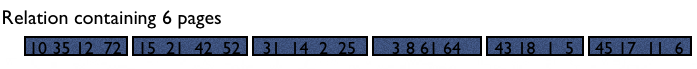• In Step 1, we will read 2 pages of R at a time, sort and then write back to disk: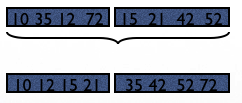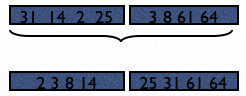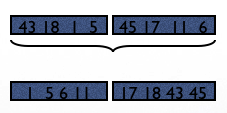• Total cost of step 1: 2*6=12 pages.
• Step 2: Now assume M=3.• Read one page from each group, continuously delete the smallest value and put it in the output buffer.• Given we can read one page from each sorted group, we can finish the sorting in one execution of Step 2.
• Total cost of step 2: 1*6 = 6
• Overall cost (steps 1 and 2): 12+6 = 18## Multi-step version of Step 2¶

• Let us see a different example.

Suppose PAGES(R)=1,000 and M = 11

• Step 1: We create 91 sorted groups (total cost 2,000 pages)

• In Step 2, we cannot really merge all 91 groups (since M=11). We have to sort and write groups.

We can merge 10 groups (and use 1 block for output) at a time.

Reduce: 91 sorted groups to 10 sorted groups

Total cost: 2,000 pages (read once, write once)

• We can repeat Step 2 to merge the remaining 10 groups and output the result.

Total cost: 1,000 pages (read once and output)

• Total cost: 5,000 pages.

• For simplicity, we can just disregard the 1 output buffer in our computations.

## Sort based duplicate removal¶

• When a “distinct” projection is needed, we can do the following:

```Sort the relation
Read the relation in sorted order
For each tuple:
If it is already seen
Else:
output
```
• Need to keep in memory the last seen tuple only, so 1 page is sufficient for the operation.

• It is possible to combine sort and duplicate removal

```Read the relation in groups, remove all unwanted attributes

Sort the tuples in memory, remove duplicates (distinct) and write
the sorted group to disk

Read the sorted group for k-way-merge, during merge remove any
```

## Sort based projection¶

• Cost is similar to external sort, but the relation being read in the second stage is reduced in size by removing unnecessary attributes
• Tuples are smaller (how many attributes are removed?)
• Duplicates are removed (how many duplicates are there?)

## Hash based projection¶

• To compute “distinct R.A, R.B,…”:

Read all tuples in R and extract the projected attributes

Hash the remaining attributes to buckets 1 … M in memory (and continuously remove duplicates from buckets in memory)

Whenever a bucket in memory is full, write it to disk

For any bucket that takes up more than one disk page, read it back from disk to memory and remove duplicates within the bucket.

• Example: 2 pages for hashing and 1 page for processing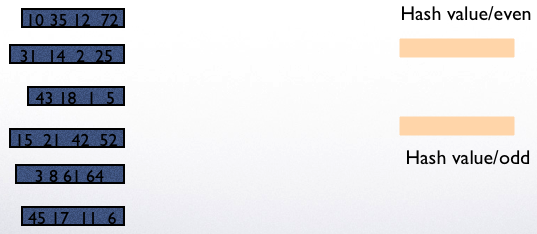• Read and put values into the two buckets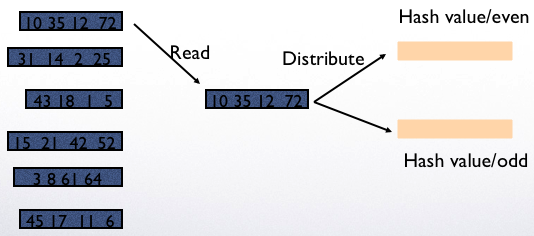• If the bucket needs to be computed before the query executes, it needs to be written to disk.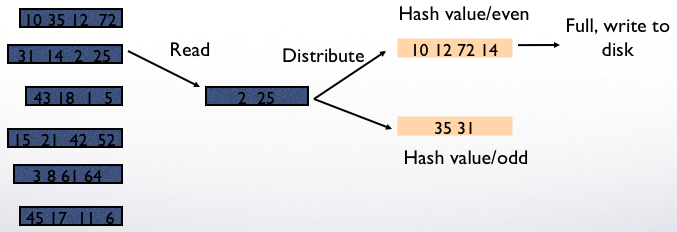• Once hashing is complete, different operations can be mapped to different buckets and applied independently in each bucket.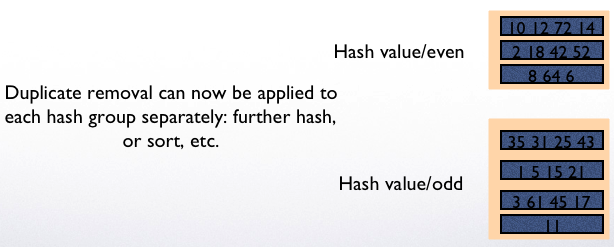## Hash based projection¶

• The cost:

The relation has to be read once for hashing

If all the buckets after reduction are too large fit in a single memory block, then the relation will be written once to disk

If all the disk pages in a single bucket will fit in the M available blocks, then the last step can be performed in one read.

## Set operations¶

• To compute A UNION B (with duplicate removal), we first hash both A and B together and remove duplicates in each bucket separately.
• To compute A - B, we hash A and B into the same buckets and then compute A - B to each bucket separately.

## Selections¶

• To compute SELECT FROM R WHERE C

[Table Scan] Read all of R one block at a time and check C. Only need a single buffer page to read R. Total cost PAGES(R).

[Index Scan] Read the index to check all or part of C, find qualifying tuples. Read those tuples from disk and return. Total cost: the cost of index scan + cost of relation scan.

• Example:

```SELECT  R.C, R.D    FROM R
WHERE R.A=10 AND R.B=5 AND R.C<30 ;
```
• Given index I1 on R.A,R.B such that

I1 has depth 3

I has about k leaf nodes with about k*c tuples in general for any specific R.A,R.B value (I.e. duplicate tuples)

• Finding the tuple identifier of all tuples with R.A=10 AND R.B=5 takes 3 + k disk reads in the average.

• We still need to read the qualifying k*c tuples from disk to check R.C, and return R.C, R.D

• Given k*c tuples with R.A=10 and R.B=5, how many disk pages for R need to be read to find all these tuples?

If the relation is not sorted/clustered with respect to R.A, R.B, then we might end up reading a different page for each single tuple. The worst case then is k*c disk reads.

If the relation is clustered with respect to R.A,R.B and suppose each disk page stores about n tuples of R, then we need to read about k*c/n disk pages.

• Given index I2 on R.A,R.B,R.C such that

I has depth 3

I has about k leaf nodes with about k*c tuples in general for any specific R.A,R.B value (I.e. duplicate tuples)

Find the leaf nodes for R.A=10 AND R.B=5. For these, we scan the siblings left to right until R.C >= 30. In the worst case 3 + k nodes are scanned.

Return all the R.C values from the index, no need to read the relation.

## Access Paths¶

• Given a query

```SELECT attrs FROM R WHERE C
```

the following are the possible access paths for this relation

• Table scan: Always possible.

• Hash index: Possible if the hash index is on a subset of attributes A1,…,An such that all conditions in C for A1,…,An are for equality and are conjunctive (ANDed).

• B-tree index with search key A1,…,Am such that a prefix A1,…,An of A1,…,Am have equality conditions in C.

• After an index scan, it is necessary to scan the relation if

• C contains conditions on attributes that are not in the index, or
• Projection attributes are not all in the index
• Example:

```FROM R WHERE R.A=10 AND R.B<10 AND R.C > 20
```
• Can we use B-tree indices on

I1 on R(A,B) ?

I2 on R(B,C) ?

I3 on R(D,A) ?

## Complex Conditions¶

• Given an index can be used to evaluate the selection condition only partially, how can we compute complex conditions?

FROM R WHERE C1 AND C2

• Use index to find tuples that satisfy C1, read tuples from disk and check C2 in memory.

• Use two indices to find tuples that satisfy C1 and C2 separately, take the intersection of the tuple identifiers.

• Given multiple indices to evaluate part of a complex query condition C, which one to choose?

• Choose the most selective one.

• If the resulting tuples can be reduced significantly by another index, use the next most selective index.

• Otherwise, do a table scan.

## Nested loop join¶

• The naïve approach R join S (R outer, S inner)

```For each one page of the outer relation (R):
read the page into 1 block of memory
for all pages of the inner relation (S):
read the page into 1 block of memory
join with the block in memory
```
• Needs only 2 blocks of memory

• For each block of R, S is read once.

S is read a total of PAGES(R) times, total blocks of S read is then PAGES(R)*PAGES(S)

R is read once, therefore the total cost is PAGES(R)+PAGES(R)*PAGES(S)

## Block nested loop join¶

• Given M buffer pages for the query

```for each M-1 block chunks of outer relation(R)
read M-1 pages R into memory
for each page of inner relation (S)
read the page into 1 memory block
join the tuples in S with all pagse of R in memory
```
• S is read a total of ceiling(PAGES(R)/(M-1)) times, total I/O cost of S is then PAGES (S)* ceiling(PAGES(R)/(M-1))

• As always, R is read once. So, the total cost is

PAGES(R) + PAGES(S)* ceiling(PAGES(R)/(M-1))

## Index nested loop join¶

• Index loop join assumes a look-up of matching tuples for S using an index.

```Given R join S on R.A=S.A

Read R one block at a time
For each tuples r of R
Use index on S(A) to find all matching tuples
Read the tuples from disk and join with
```
• If R is not sorted on A, then we might end up reading the same tuples of S many times

• What if A is R’s primary key?

Then, for each A value, we will look up S only once

• Cost:

• The outer relation R is read once, PAGES(R)

• Assume for every tuple r in R, there are about c tuples in S that would join with R (ideal case is c is very small or 0 most of the time)

• Then, for each tuples in R: - We find the matching tuples in S (cost is index look-up, in the

best case one tuple for each level, so h+1 for an index with h levels)

• Then, if we need attributes that are not in the join, we read c tuples from S (which can be in c pages in the worst case)
• Total cost is between PAGES(R) + TUPLES(R) * (h+1) (if no tuples match or index only scan is fine) and PAGES(R) + TUPLES(R) * (h+1+c)
• Example:

```SELECT S.B FROM R,S WHERE R.A = S.B

Index I1 on S(B) with 2 levels (root, internal, leaf)
PAGES(R)=100, TUPLES(R)= 2000
PAGES(S)=200, TUPLES(S)= 4000

Cost = 100 (for reading R) + 2000*3 (index look up for each tuple of R)

SELECT S.C  FROM R,S WHERE R.A = S.B

Assume statistics are same as above, but we now need to reach
each matching tuple (at most 4000 tuples will match)

Cost = 100+2000*3+4000 (one page read for each matched tuple)
```

How could this be, there are only 200 pages of S?

Well, if we are not finding all pages that we need to read first and reading each page as we find a match, we may end up reading the pages for S multiple times.

Of course, in reality, you will likely do a lot of reduction of duplicate page requests in memory and improve on this. This is the worst case scenario.

Will we ever do this?

No, we will choose not to use index join for this case, clearly it looks very expensive and we better do some other operation.

• Another example:

```SELECT S.B FROM R,S WHERE R.A = S.B AND R.B=100

Index I1 on S(B) with 2 levels (root, internal, leaf)
PAGES(R)=100, TUPLES(R)= 2000
PAGES(S)=200, TUPLES(S)= 4000
Suppose only 3 tuples match R.B=100

So, we can:
Scan all of R to find these 3 tuples (100 pages)
Read matching tuples from S (3*3)
Total cost = 109 (using only 2 pages of memory)

This would cost a lot more in block-nested loop join
with M=2 (200 pages). So, index join is for cases
where the outer relation is very small (normally after
a selection)
```

## Sort-merge join¶

• Sort both R and S first

• Read R and S one block at a time, and join the matching tuples.

• Sort merge is similar to Step 2 of the sorting algorithm.

• Sort-merge join

• Example:

```R: [1, 2] [5, 8]    S:[1,5] [6, 7]
Read [1,2] and [1,5] first, join 1.
Read the next block of R, [5,8] [1,5]. Join 5
Read the next block of S, [5,8] [6,7]. No more tuples, done.
```
• If each joining attribute has unique values in R and S, then the join can be performed in a single step without reading each relation once forward, PAGES(R) + PAGES(S) + the cost of sort

• If there are duplicate values, then we must worry about if all the duplicate values from both relations will fit in memory

• Example:

```R [1,2] [2,2] [2,2] [2,2] [2,2] [2,2] [2,2] [2,2] [2,2][2,3]
S [1,1] [1,1] [1,1][2,2] [2,2] [2,2] [2,2] [2,2][3,4]
```
• We need a total of 15 buffer pages to be able to compute this join at one step.

• In general, a block-nested loop join is performed for duplicate values.

## Hash join¶

• Hash both R and S on the joining attribute, read R and S once:

PAGES(R) + PAGES(S)

• Each bucket will contain both tuples of R and S

• Read each bucket into memory to perform the join within the bucket

• If a bucket cannot fit in memory, then other methods should be used to perform the join operation within the bucket

## Summary¶

• Sorting and hashing are two main methods that can be used to implement other operators.
• In particular, sorting may help reduce the cost of multiple operations upstream